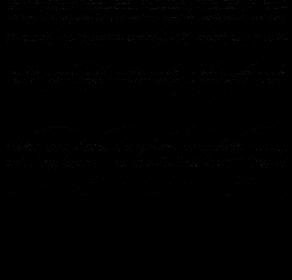# How to properly manipulate pixel value in image using SOIL library in OpenGL ?

Hi, I’m trying to load image using SOIL library and do some pixel manipulation, but the output on my window in black like broken tv picture. Like thisHere’s how I call using SOIL :

``````  image = SOIL_load_image("Images/image1.png", &width, &height, 0, SOIL_LOAD_RGBA);
if(image == NULL) exit(0);
``````

And here’s my pixel manipulation function :

``````static void genImages()
{
unsigned char *p, *p1, r, g, b;
float R, G, B, L, M, S;

if(image1 == NULL) {
image1 = (unsigned char *)malloc(width*height*4);
if(image1 == NULL) exit(0);
}

p = image;
r = *(p+0);
g = *(p+1);
b = *(p+2);

p1 = image1;
R = *(p1+0);
G = *(p1+1);
B = *(p1+2);

for (int i = 0; i < width; i++) {
for (int j = 0; j < height; j++) {

L = ( 7.88 * r) + ( 3.51 * g) + ( 4.11 * b) ;
M = ( 3.45 * r) + ( 7.15 * g) + ( 3.86 * b) ;
S = ( 0.03 * r) + ( 0.18 * g) + ( 1.46 * b) ;

R = (  0.0809 * L) + ( -0.1305 * M) + (  0.1167 * S);
G = ( -0.0102 * L) + (  0.0540 * M) + ( -0.1136 * S);
B = ( -0.0004 * L) + ( -0.0041 * M) + (  0.6935 * S);

}
p += 4;
p1 += 4;
}
}
``````

I can make sure my rendering is correct because I use the same working rendering before, I just change function to call.
But here’s how I call it :

``````    genImages();
argDrawMode2D(vp);
argDrawImage(image1);
argSwapBuffers();
glutPostRedisplay();
``````

So, I guess my problem is in my function. Any advice ?

Learn to program in C.

You copy pixels from image1 into the variables R, G and B but never use those values. Then you store the results of calculations in R, G and B and never use those values either. And you never store anything in image1.

This has nothing to do with OpenGL and is off-topic for this board.

[QUOTE=GClements;398203]Learn to program in C.

You copy pixels from image1 into the variables R, G and B but never use those values. Then you store the results of calculations in R, G and B and never use those values either. And you never store anything in image1.

This has nothing to do with OpenGL and is off-topic for this board.[/QUOTE]

Thank you. My bad, I’m moving from OpenCV and C++, I’ve done this manipulation in C++ and OpenCV works perfectly with Mat. Now have limited environment and required to use OpenGL and C. Also rgb is value from “Image” (original file) and I believe I save RGB to Image1. Image1 is generated image, copy image, the one I want to display so I dont have to manipulate the original image.

I’ve updated the loop :

`````` for (int i = 0; i < width; i++) {
for (int j = 0; j < height; j++) {

L = (17.8824 * (*(p+0))) + (43.5161 * (*(p+1))) + ( 4.1194 * (*(p+2))) ;
M = ( 3.4557 * (*(p+0))) + (27.1554 * (*(p+1))) + ( 3.8671 * (*(p+2))) ;
S = ( 0.0300 * (*(p+0))) + ( 0.1843 * (*(p+1))) + ( 1.4671 * (*(p+2))) ;

l = 0 * L + 2.0234 * M +  -2.5258 * S;
m = 0 * L +      1 * M +       0 * S;
s = 0 * L +      0 * M +       1 * S;

*(p1+0) = (  0.0809 * l) + ( -0.1305 * m) + (  0.1167 * s);
*(p1+1) = ( -0.0102 * l) + (  0.0540 * m) + ( -0.1136 * s);
*(p1+2) = ( -0.0004 * l) + ( -0.0041 * m) + (  0.6935 * s);
``````

[QUOTE=GClements;398203]Learn to program in C.

You copy pixels from image1 into the variables R, G and B but never use those values. Then you store the results of calculations in R, G and B and never use those values either. And you never store anything in image1.

This has nothing to do with OpenGL and is off-topic for this board.[/QUOTE]

GOOD LORD. It’s working now !!
Thank you very much ! I know i’m not dumb, i’m just panicking a lot and maybe need a boost. Your comment gave me a hint and really helped me, of course I will learn to program in C harder as well. Again, thank you.

In C++, you could declare R,G,B as references ([var]unsigned char&[/var]) and that code would work. But C doesn’t have references.